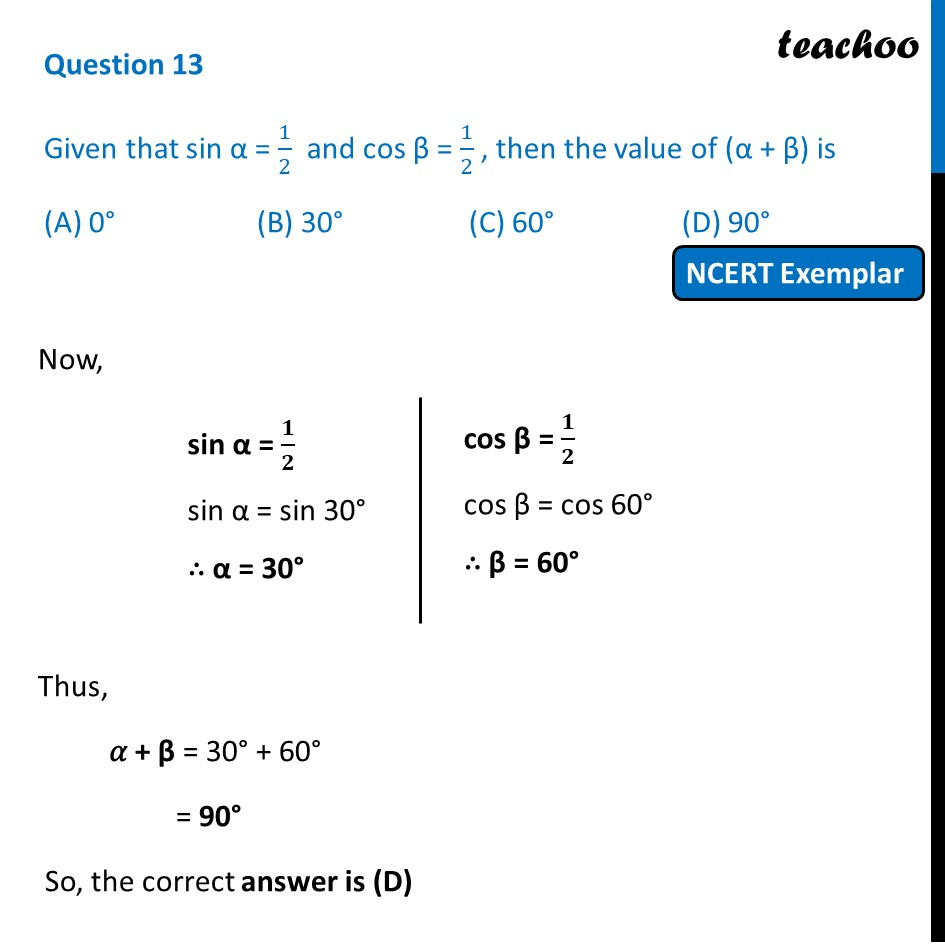## (A) 0°   (B) 30°   (C) 60°   (D) 90°1. Chapter 8 Class 10 Introduction to Trignometry (Term 1)
2. Serial order wise
3. NCERT Exemplar - MCQ

Transcript

Question 13 Given that sin α = 1/2 and cos β = 1/2 , then the value of (α + β) is (A) 0° (B) 30° (C) 60° (D) 90° Now, sin α = 𝟏/𝟐 sin α = sin 30° ∴ α = 30° cos β = 𝟏/𝟐 cos β = cos 60° ∴ β = 60° Thus, 𝛼 + β = 30° + 60° = 90° So, the correct answer is (D)